## Excel VARPA function

The VARPA function calculates the variance based on the entire population.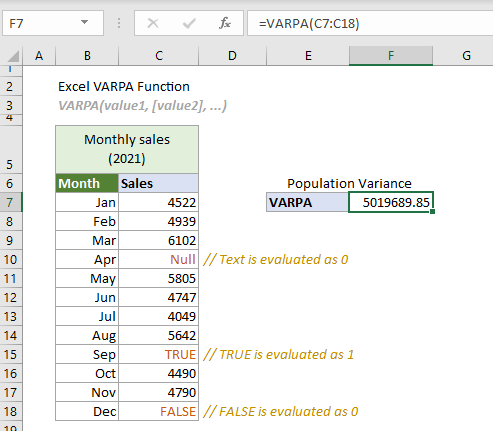#### VARPA function vs. VAR.P function

Both the VARPA function and VAR.P function can be used to estimate the variance based on the entire population. The difference between them is that the VARPA function evaluates text values and logical values in references, whereas VAR.P function does not include text values and logical values in references as a part of the calculation.

#### Excel variance functions

The following table lists all Excel variance functions and their usage notes, which can help you choose the one that best suits your needs.

 Name Data type Text and logicals in references VAR Sample Ignored VAR.S Sample Ignored VARA Sample Evaluated VARP Population Ignore VAR.P Population Ignored VARPA Population Evaluated

#### Syntax

VARP(value1, [value2], ...)

#### Arguments

• Value1 (required): The first number or reference used in the calculation.
• Value1, ... (required): The second and more numbers or references used in the calculation.

#### Remarks

1. There can be up to 255 arguments at a time.
2. Arguments can be either of:
-- Numbers;
-- Range names, arrays or cell references that contain numbers.
3. The VARPA function takes the arguments as the entire population.
Tips: If the data you provided represents a sample of the population, it is recommended to use the VARA function.
4. Logical values and text representations of numbers that you directly type in the arguments will be included in the calculation:
-- Logical values: TRUE (1) or FALSE (0);
-- Text representations of numbers: Numbers enclosed in double quotation marks such as “2”.
5.The VARAP function evaluates logical values and text values in references.
-- Logical values: TRUE is evaluated as 1, and FALSE is evaluated as 0.
-- Text value: is evaluated as 0.
Tips: If you don’t want to include logical values and text values in references as part of the calculation, it is recommended to use the VAR.P function.
6. Empty cells will be ignored in an array or cell reference.
7. The #VALUE! error occurs if any of the supplied arguments you typed directly in the function are text strings that cannot be interpreted as numbers by Excel.
8. The #DIV/0! error occurs if all supplied arguments are non-numeric.
9. The equation of the VARPA function is as follows: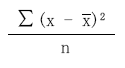Where
-- x represents each value in the sample.
--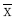is the average of the sample.
-- n is the number of cells in the sample range.

#### Return value

It returns a numeric value.

#### Example

The following table lists the monthly sales for 2021. Assuming that the monthly sales are the entire population. To calculate the variance of this entire population with the logical values and text value estimated by Excel, the VARPA function can help.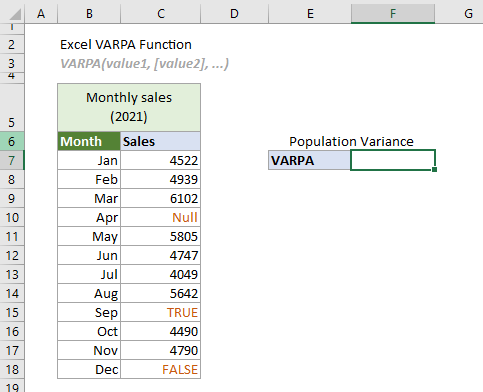Select a cell (says F7 in this case), enter the formula below and press the Enter key to get the result.

=VARPA(C7:C18)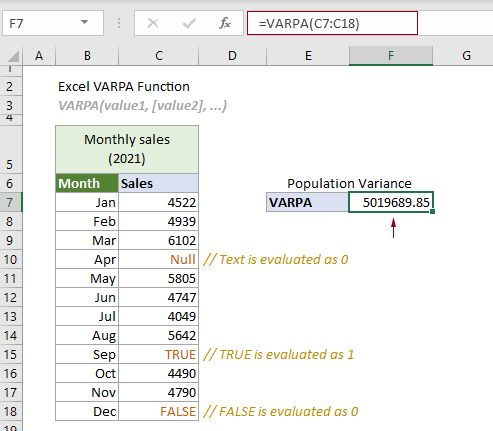Notes:

1. Arguments in the formula above are supplied as a cell range.

1) The logical value “TRUE” in the reference is evaluated as 1.
2) The logical value “FALSE” and the text “Null” in the reference is evaluated as 0.
3) The formula can be changed to:
=VARPA(C7,C8,C9,C10,C11,C12,C13,C14,C15,C16.C17,C18)
=VARPA(4522,4939,6102,0,5805,4747,4049,5642,1,4490,4790,0)

2. In this case, if you apply the VAR.P function to estimate the variance of the entire population in C6:C13, the logical values and the text in the reference will be ignored. See the following screenshot: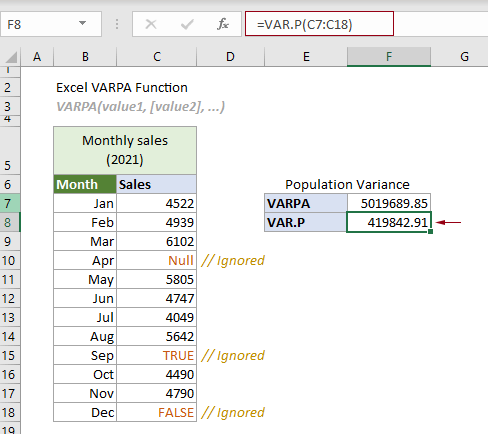#### Related Functions

Excel VARA function
The VARA function calculates the variance based on a given sample.

Excel VAR function
The VAR function calculates the variance based on a given sample.

Excel VAR.S function
The VAR.S function calculates the variance based on a given sample.

Excel VAR.P function
The VAR.P function calculates the variance based on the entire population.

Excel VARP function
The VARP function calculates the variance based on the entire population.

### The Best Office Productivity Tools

#### Kutools for Excel - Helps You To Stand Out From Crowd

 Popular Features: Find, Highlight or Identify Duplicates  |  Delete Blank Rows  |  Combine Columns or Cells without Losing Data  |  Round without Formula ... Super VLookup: Multiple Criteria  |  Multiple Value  |  Across Multi-Sheets  |  Fuzzy Lookup... Adv. Drop-down List: Easy Drop Down List  |  Dependent Drop Down List  |  Multi-select Drop Down List... Column Manager: Add a Specific Number of Columns  |  Move Columns  |  Toggle Visibility Status of Hidden Columns  |  Compare Columns to Select Same & Different Cells ... Featured Features: Grid Focus  |  Design View  |  Big Formula Bar  |  Workbook & Sheet Manager | Resource Library (Auto Text)  |  Date Picker  |  Combine Worksheets  |  Encrypt/Decrypt Cells  |  Send Emails by List  |  Super Filter  |  Special Filter (filter bold/italic/strikethrough...) ... Top 15 Toolsets:  12 Text Tools (Add Text, Remove Characters ...)  |  50+ Chart Types (Gantt Chart ...)  |  40+ Practical Formulas (Calculate age based on birthday ...)  |  19 Insertion Tools (Insert QR Code, Insert Picture from Path ...)  |  12 Conversion Tools (Numbers to Words, Currency Conversion ...)  |  7 Merge & Split Tools (Advanced Combine Rows, Split Excel Cells ...)  |  ... and more

Kutools for Excel Boasts Over 300 Features, Ensuring That What You Need is Just A Click Away...#### Office Tab - Enable Tabbed Reading and Editing in Microsoft Office (include Excel)

• One second to switch between dozens of open documents!
• Reduce hundreds of mouse clicks for you every day, say goodbye to mouse hand.
• Increases your productivity by 50% when viewing and editing multiple documents.
• Brings Efficient Tabs to Office (include Excel), Just Like Chrome, Edge and Firefox.